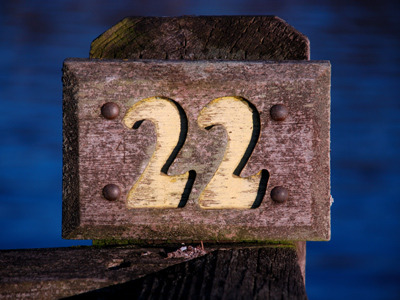What is 22% written as a fraction?

# Converting Percentages to Fractions

This Math quiz is called 'Converting Percentages to Fractions' and it has been written by teachers to help you if you are studying the subject at middle school. Playing educational quizzes is a fabulous way to learn if you are in the 6th, 7th or 8th grade - aged 11 to 14.

It costs only \$12.50 per month to play this quiz and over 3,500 others that help you with your school work. You can subscribe on the page at Join Us

To convert a percentage to a fraction we must eliminate the “%” symbol by moving it two spaces to the left of the given number. In this case the given number is “12”. So, if you have 12%, the “%” is replaced with a decimal point that is then moved two spaces to the left of the 12 to give you: .12. The decimal point represents the number “100”. We then put the percentage number (12) as the numerator in a fraction and the “100” becomes the denominator. This converts 12% to look like 12/100.

It is important to remember that the “%” symbol always represents the number “100”.

1.
33% = ___
33/1000
33/10
33/100
3/300
33% converts to the decimal of .33. We then put the percentage number “33” as the numerator and the number “100” as the denominator and we get 33/100. Answer (c) is the correct answer
2.
64% = ___
6/400
64/100
64/10
64/1000
64% converts to the decimal of .64. We then put the percentage number “64” as the numerator and the number “100” as the denominator and we get 64/100. Answer (b) is the correct answer
3.
119% = ___
11/900
119/10
1/19
1 19100
119% converts to the decimal of 1.19. Remember, the decimal point moves two spaces to the left. This means the decimal point will come up between the two “1s”. We then put the percentage number “119” as the numerator and the number “100” as the denominator and we get 119/100 which can then be simplified as 1 19100. Answer (d) is the correct answer
4.
87% = ___
87/100
87/1000
87/10
87/001
87% converts to the decimal of .87. We then put the percentage number “87” as the numerator and the number “100” as the denominator and we get 87/100. Answer (a) is the correct answer
5.
39% = ___
39/10
3/900
39/100
3/.09
39% converts to the decimal of .39. We then put the percentage number “39” as the numerator and the number “100” as the denominator and we get 39/100. Answer (c) is the correct answer
6.
71% = ___
71/1
71/100
71/1000
71/10
71% converts to the decimal of .71. We then put the percentage number “71” as the numerator and the number “100” as the denominator and we get 71/100. Answer (b) is the correct answer
7.
53% = ___
53/1
1/53
5/300
53/100
53% converts to the decimal of .53. We then put the percentage number “53” as the numerator and the number “100” as the denominator and we get 53/100. Answer (d) is the correct answer
8.
102% = ___
102/10
10/200
102/1000
1 150
102% converts to the decimal of 1.02. Remember, the decimal point moves two spaces to the left. This means the decimal point will come up between the “1” and the “0”. We then put the percentage number “102” as the numerator and the number “100” as the denominator and we get 102/100 which can then be simplified as 1 2100 or 1 150. Answer (d) is the correct answer
9.
88% = ___
88/1000
88/10
88/100
8/800
88% converts to the decimal of .88. We then put the percentage number “88” as the numerator and the number “100” as the denominator and we get 88/100. Answer (c) is the correct answer
10.
22% = ___
22/100
22/1
22/10
22/001
22% converts to the decimal of .22. We then put the percentage number “22” as the numerator and the number “100” as the denominator and we get 22/100. Answer (a) is the correct answer
Author:  Christine G. Broome